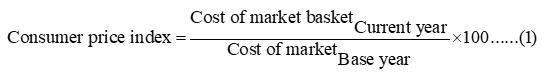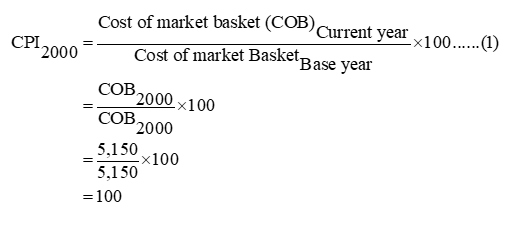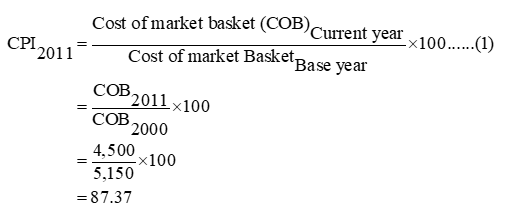# The table shows the cost of a fixed basket of goods that a typical urban consumer would buy in the economy of Kindleberger. The base period for the consumer price index (CPI) is the year 2000. Please specify answers to two decimal places.YearCost of a basket of goods2000\$5,150.002011\$4,500.002012\$7,725.00What is the CPI for 2000?CPI for 2000:  What is the CPI for 2011?CPI for 2011:  What is the CPI for 2012?CPI for 2012:

Question
143 views

The table shows the cost of a fixed basket of goods that a typical urban consumer would buy in the economy of Kindleberger. The base period for the consumer price index (CPI) is the year 2000. Please specify answers to two decimal places.

Year Cost of a basket of goods
2000 \$5,150.00
2011 \$4,500.00
2012 \$7,725.00

What is the CPI for 2000?

CPI for 2000:

What is the CPI for 2011?
CPI for 2011:

What is the CPI for 2012?
CPI for 2012:

check_circle

Step 1

Consumer price index refers to the changes in the price level of consumer goods and services purchased by households.

Step 2

The equation for calculating the consumer price index is given below.Substitute respective values in equation (1) to calculate the consumer price index for different years.The calculated value shows that the consumer price index for the base year is 100.

Step 3

The consumer price index for the year 2011 can be calculated as follows.The calculated value shows that the consumer price index of the year 2011 is 87.37.

...

### Want to see the full answer?

See Solution

#### Want to see this answer and more?

Solutions are written by subject experts who are available 24/7. Questions are typically answered within 1 hour.*

See Solution
*Response times may vary by subject and question.
Tagged in